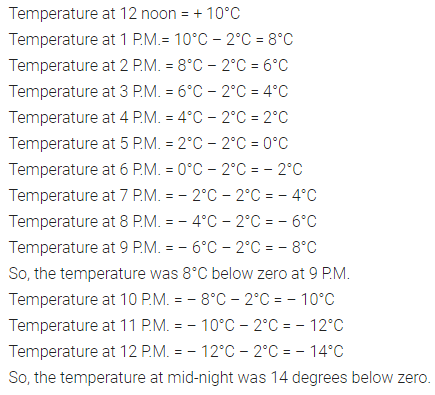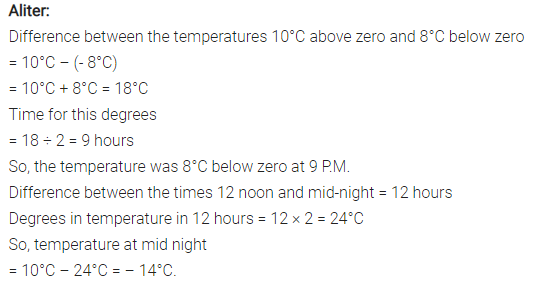# The temperature at 12 noon was 10°C above zero. If it decreases

The temperature at 12 noon was 10°C above zero. If it decreases at the rate of 2°C per hour until mid-night, at what time would the temperature be 8°C degrees below zero? What would be the temperature at mid night?